﻿ Java - Shape with the getArea() method

# Java Interface: Shape with the getArea() method, implement the Shape interface

## Java Interface: Exercise-1 with Solution

Write a Java program to create an interface Shape with the getArea() method. Create three classes Rectangle, Circle, and Triangle that implement the Shape interface. Implement the getArea() method for each of the three classes.

Sample Solution:

Java Code:

``````// Shape.java
// This is an interface named 'Shape' that defines a contract for classes to implement.
public interface Shape {
// Abstract method signature for getting the area of a shape.
double getArea();
}
```
```
``````// Rectangle.java
// This is the 'Rectangle' class that implements the 'Shape' interface.

public class Rectangle implements Shape {
// Private instance variables to store the dimensions of the rectangle.
private double length;
private double width;

// Constructor for creating a Rectangle object with given length and width.
public Rectangle(double length, double width) {
this.length = length;
this.width = width;
}

// Implementation of the 'getArea' method as required by the 'Shape' interface.
@Override
public double getArea() {
// Calculate and return the area of the rectangle.
return length * width;
}
}
``````
``````// Circle.java
// This is the 'Circle' class that implements the 'Shape' interface.

public class Circle implements Shape {
// Private instance variable to store the radius of the circle.

// Constructor for creating a Circle object with a given radius.
}

// Implementation of the 'getArea' method as required by the 'Shape' interface.
@Override
public double getArea() {
// Calculate and return the area of the circle using the formula: π * r^2.
}
}
``````
``````// Triangle.java
// This is the 'Triangle' class that implements the 'Shape' interface.

public class Triangle implements Shape {
// Private instance variables to store the base and height of the triangle.
private double base;
private double height;

// Constructor for creating a Triangle object with a given base and height.
public Triangle(double base, double height) {
this.base = base;
this.height = height;
}

// Implementation of the 'getArea' method as required by the 'Shape' interface.
@Override
public double getArea() {
// Calculate and return the area of the triangle using the formula: 0.5 * base * height.
return 0.5 * base * height;
}
}
``````
``````// Main.java
// Main class that creates objects of Rectangle, Circle, and Triangle

public class Main {
public static void main(String[] args) {
// Create a Rectangle object with length=10 and width=12
Rectangle rectangle = new Rectangle(10, 12);

// Create a Circle object with radius=3
Circle circle = new Circle(3);

// Create a Triangle object with base=4 and height=6
Triangle triangle = new Triangle(4, 6);

// Print the area of the Rectangle
System.out.println("Area of the Rectangle: " + rectangle.getArea());

// Print the area of the Circle
System.out.println("Area of the Circle: " + circle.getArea());

// Print the area of the Triangle
System.out.println("Area of the Triangle: " + triangle.getArea());
}
}
``````

Sample Output:

```Area of the Rectangle: 120.0
Area of the Circle: 28.274333882308138
Area of the Triangle: 12.0
```

Flowchart of Interface Shape: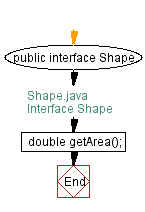Flowchart of Class Rectangle: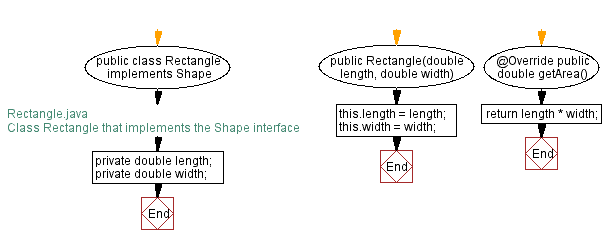Flowchart of Class Circle: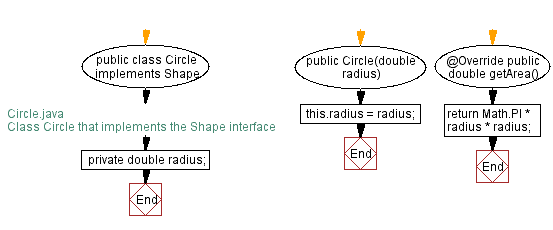Flowchart of Class Triangle: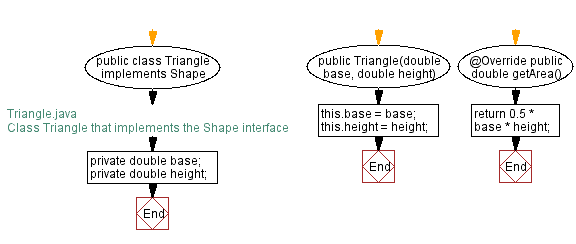Flowchart of Main Class: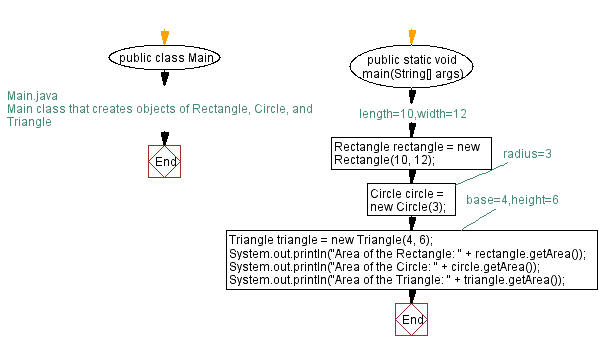Java Code Editor:

What is the difficulty level of this exercise?

﻿